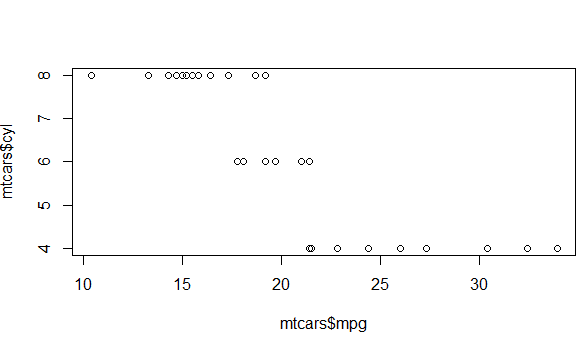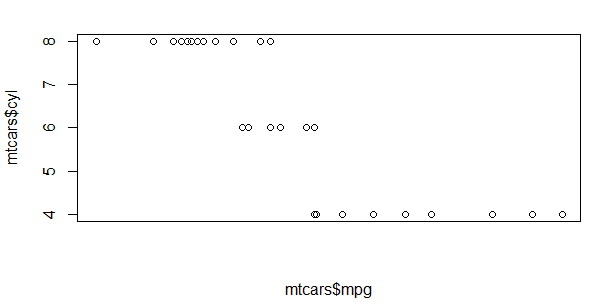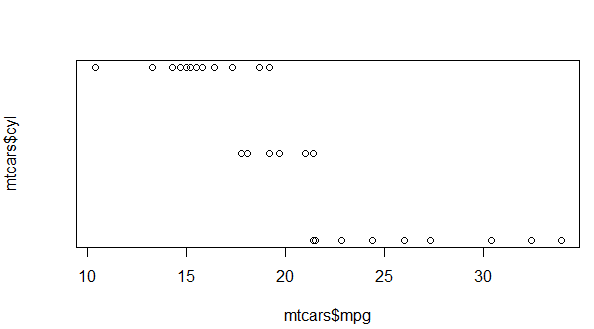# Remove plot axis values

0 votes
1 view

I was just wondering if there is a way to get rid of axis values, either the x-axis or y-axis respectively, in an r-plot graph.

I know that axes = false will get rid of the entire axis, but I would only like to get rid of the numbering.

## 1 Answer

0 votes
by (25.4k points)

To remove x and y-axis values use xaxt="n" and yaxt="n"  that suppress the x and y-axis respectively.

For example:

For the plot below:

data(mtcars)

plot(x= mtcars\$mpg,y=mtcars\$cyl)To remove x and y-axis values:

plot(x= mtcars\$mpg,y=mtcars\$cyl, xaxt='n')

plot(x= mtcars\$mpg,y=mtcars\$cyl, yaxt='n')

Outputs:0 votes
1 answer
+1 vote
2 answers
0 votes
1 answer
0 votes
1 answer
0 votes
1 answer